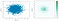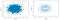# My Understanding of Exploratory Data Analysis

Exploratory data analysis (EDA), pioneered by John Tukey, set a foundation for the field of data science. The key idea of EDA is that the first and most important step in any project based on data is to look at the data. By summarizing and visualizing the data, you can gain valuable intuition and understanding of the project. Exploratory analysis should be a cornerstone of any data science project.

# Part 1: Location Estimator

It is defined as the average of all values after dropping a fixed number of extreme values.

x has been sorted in the formula. A trimmed mean eliminates the influence of extreme values.

Weighted mean/median

It is the sum of all values times a weight divided by the sum of the weights. There are two reasons in favor of weighted mean/median:

• Some values are intrinsically more variable than others, and highly variable observations are given a lower weight. For example, if we are taking the average from multiple sensors and one of the sensors is less accurate, then we might down-weight the data from that sensor.
• The data collected does not equally represent the different groups that we are interested in measuring. For example, because of the way an online experiment was conducted, we may not have a set of data that accurately reflects all groups in the user base. To correct that, we can give a higher weight to the values from the groups that were underrepresented.

Weighted median is calculated in this way: instead of the middle number, the weighted median is a value such that the sum of the weights is equal for the lower and upper halves of the sorted list. Like the median, the weighted median is robust to outliers.

The implementation is as follows (use Python package `wquantiles` and `numpy`):

`np.average(state['Murder.Rate'], weights=state['Population'])wquantiles.median(state['Murder.Rate'], weights=state['Population'])`

Summary

• The basic metric is the mean.
• While robust estimators (median, trimmed mean, weighted mean/median) are valid for small data sets, they do not provide added benefit for large or even moderately sized data sets.
• Statisticians and data scientists use different terms for the same thing. Statisticians use the term estimate while data scientists use the term metric for location statistics.
• location and variability are referred to as the first and second moments of a distribution. The third and fourth moments are called skewness and kurtosis. Skewness refers to whether the data is skewed to larger or smaller values; kurtosis indicates the propensity of the data to have extreme values.

# Part 2: Variability Estimator

Variance: the sum of squared deviations from the mean divided by n — 1 where n is the number of data values. The reason why we use n-1 is because we can get an unbiased estimate of variance and standard deviation. There are n — 1 degrees of freedom since there is one constraint: the standard deviation depends on calculating the sample mean. For most problems, data
scientists do not need to worry about degrees of freedom.

Mean absolute deviation: the mean of the absolute values of the deviations from the mean. It is also called l1-norm, Manhattan norm

Median absolute deviation from the median (MAD): the median of the absolute values of the deviations from the median. Normally, we need to normalize MAD by a multiplication factor 1.4826, which means that 50% of the normal distribution fall within the range +/- MAD. See statsmodels.robust.scale.mad

`from statsmodels import robustdata = np.random.randn(30, 2)df = pd.DataFrame(data, columns=['column_1','column_2'])print(df['column_1'].std())print(robust.scale.mad(df['column_1']))`

Range: the difference between the largest and the smallest value in the data set.

Order statistics: metrics based on the data values sorted from smallest to biggest.

Percentile and quantile

Interquartile Range (IQR): the difference between 25th percentile and the 75th percentile.

`data = np.random.randn(30, 2)df = pd.DataFrame(data, columns=['column_1','column_2'])df.head(3)df['column_1'].quantile(0.75)-df['column_1'].quantile(0.25)`

Percentiles

`df['column_1'].quantile([0.05, 0.75, 0.95])`

# Part 3: Distribution Estimator

• horizontal thick line in the box: median
• top/bottom horizontal thin line in : 75th and 25th percentiles
• the dashed lines are called whiskers, and it will extend to the furthest point within 1.5 times the Interquartile Range (IQR). Any data outside of the whiskers is plotted as single points or circles (often considered as outliers).
• range: the y-coordinate range
`df['column_1'].plot.box()`

An improved version of boxplots is violinplot:

`sns.violinplot(df['column_1'], inner='quartile')`

The density is mirrored and flipped over, and the resulting shape is filled in, creating an image resembling a violin. The advantage of a violin plot is that it can show nuances in the distribution that aren’t perceptible in a boxplot. On the other hand, the boxplot more clearly shows the outliers in the data.

Frequency table and histogram

`binned_column = pd.cut(df['column_1'], 10)print(type(binned_column))binned_column.value_counts(sort=False)`

A histogram is a way to visualize the frequency table.

`ax = df['column_1'].plot.hist(figsize=(6, 6))ax.set_xlabel('range value')`

Density plots and estimates

A density plot is a smoothed version of a histogram.

`ax = df['column_1'].plot.hist(figsize=(6, 6),bins=11)df['column_1'].plot.density(ax=ax)ax.set_xlabel('range value')`

We can also use bar/pie plot to show the categorical data:

`ax=df['column_1'].plot.bar()/pie()ax.set_xlabel('')ax.set_ylabel('')`

For categorical data, the mode is the value that appears most often in the data, and it, however, cannot be used for numeric data.

# Part 4: Correlation

`print(df.corr())sns.heatmap(df.corr())`

Like the mean and standard deviation, the correlation coefficient is sensitive to outliers in the data.

Another way of visualizing the relationship between two measured data variables is with a scatterplot. The x-axis represents one variable and y-axis another, and each point on the graph is a record.

`df.plot.scatter(x='column_1', y='column_2')`

However, scatter plot might not be proper for data sets that contain a large amount of records. In this case, a better option is to use a hexagonal binning plot. Rather than plotting points, which would appear as a monolithic dark cloud, we grouped the records into hexagonal bins and plotted the hexagons with a color indicating the number of records in that bin.left: scatter; right: hexagonal binning
`df.plot.hexbin(x='column_1', y='column_2')`

An alternative is to use contour plot: the contours are essentially a topographical map to two variables; each contour band represents a specific density of points, increasing as one nears a “peak.”left: scatter; right: contour

In a summary, in order to show the correlation between two numerical variables, we have the following solutions:

• scatter plot: it is often used when we have small amount of data records
• hexagonal binning and contour plot: they are used when there are a lot of data records
• heat map: it is often used to illustrate matrix. For example, correlation matrix, confusion matrix and so on

# Part 5: Contingency Table

`import pandas import numpy   # creating some data a = numpy.array(["foo", "foo", "foo", "foo",                  "bar", "bar", "bar", "bar",                  "foo", "foo", "foo"],                 dtype=object)   b = numpy.array(["one", "one", "one", "two",                  "one", "one", "one", "two",                  "two", "two", "one"],                 dtype=object)   res = pandas.crosstab(a, [b], rownames=['a'], colnames=['b']) res`

# Part 6: More Variables (more than 2)

`airline_stats.boxplot(by='airline', column='pct_carrier_delay')sns.violinplot(airline_stats['airline'], airline_stats['pct_carrier_delay'])`

The same with scatter plots, hexagonal binning plots and so on. The basic idea is conditioning variables in a graphics system, and showing pairs of variables sequentially.

`loans_income = pd.read_csv(LOANS_INCOME_CSV, squeeze=True)sample_data = pd.DataFrame({    'income': loans_income.sample(1000),    'type': 'Data',})sample_mean_05 = pd.DataFrame({    'income': [loans_income.sample(5).mean() for _ in range(1000)],    'type': 'Mean of 5',})sample_mean_20 = pd.DataFrame({    'income': [loans_income.sample(20).mean() for _ in range(1000)],    'type': 'Mean of 20',})results = pd.concat([sample_data, sample_mean_05, sample_mean_20]) g = sns.FacetGrid(results, col='type', col_wrap=1,                   height=2, aspect=2)g.map(plt.hist, 'income', range=[0, 200000], bins=40)g.set_axis_labels('Income', 'Count')g.set_titles('{col_name}')plt.tight_layout()plt.show()`

# Part 7: Reference

• Practical Statistics for Data Scientists 50+ Essential Concepts Using R and Python: CHAPTER 1 Exploratory Data Analysis

Code